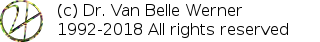Home Papers Reports Projects Code Fragments Dissertations Presentations Posters Proposals Lectures given Course notes

# Estimating the phase of a subbin sine from a local group of frequencies.

Werner Van Belle1 - werner@yellowcouch.org, werner.van.belle@gmail.com

1- Yellowcouch;

Abstract :  When sinusoidal modelling a peak from a fourier spectrum, the phase must often be estimated. Under specific FFT windows these phases can be interpolated. However, in the general sense such interpolation techniques do not work very well. We present a general purpose solution to estimate the frequency of a wave by looking at its neighboring bins.

Keywords:  sinusoidal modelling, phase estimation
Reference:  Werner Van Belle; Estimating the phase of a subbin sine from a local group of frequencies.; Audio Processing; YellowCouch; January 2014

When a distinctive sine wave is present in a signal it will show up in the Fourier analysis as a peak. This peak will generally cover multiple bins due to an incorrect alignment towards the bin. Assume that the actual sine wave is at bin k (a non integer value), how can we then, in a general purpose sense, estimate the phase of the wave by looking at the bins surrounding the peak ?

To obtain that information an easy solution exists: generate the wave and integrate it towards the signal. The resulting integral phi will be a complex value that specifies the angle under which the integral is optimal.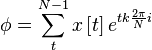is the equation to do that. Thereby x is the signal representation in the time domain and k is the exact bin position (not necessarily an integer). n is the size of the modulation window.

The above can easily be obtained by scanning the entire window and simply calculating the result. It requires O(n) operations, which is a bit too expensive if we need to do this for many peaks in the spectrum.

Let's see whether we can obtain similar phase information from the neighborhood of the associated peak in the spectrum. To go there we expand x[t] into its Fourier representation.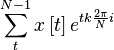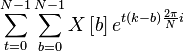Here X[b] is the Fourier representation. Swapping the summations gives us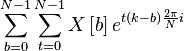which allows us to move X[b] upwards.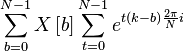which shows us that the integrand between the exact sine and the signal is a summation of the values of the Fourier bins with a certain constant C, defined as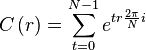when r=k-b the integral thus becomes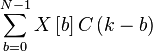If we calculate C and store them in a small lookup table then we can directly assess the phase of a sine wave at bin k, by integrating the neighborhood of the peak in the spectrum. Generally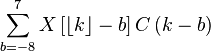is sufficiently close to be appreciably correct and it requires only 16 operations per peak.

Of course, C can also be calculated without a need to go through a full summation of the window size. To do so we look into the continuous integral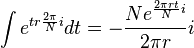Given the bounds [0:N] we get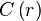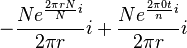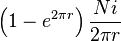When r=0, then the value should be obtained as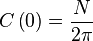This equation work also for the discrete version, and when used solely to obtain the angle phi, we can multiplyby 2 pi/N, which leads to the following equation for the phase of the sine at bin k: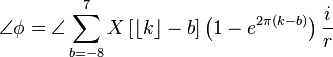http://werner.yellowcouch.org/werner@yellowcouch.org